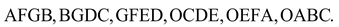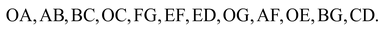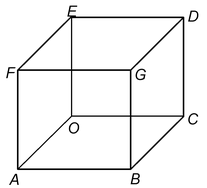# SOLID FIGURES

## Surface Area and Volume of Class 9

If any figure such as cuboids, which has three dimensions length, width and height are known as three dimensional figures. Where are rectangle has only two dimensions i.e. length and width. Three dimensional figures have volume in addition to areas of surface from which these solid figures are formed.

## CUBOID:

A cuboid is a solid figure, held by six rectangular plane regions

Here, In cuboid we have six faces namelyWe also have 12 edges, where two sides meets namely### CUBE:

A cuboid in which we have all length, breadth, height of equal lengths, is called a cube.It also has six faces and twelve edges.